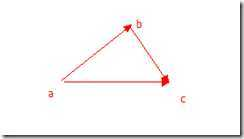# 数学趣味----抽象

1.问题：将下面每一组4个数经过加减乘除得到24.（是不是看上去很简单哦）

` (1)5,5,5,1;    (2)3,3,7,7;`
` (3)4,4,7,7;    (4)3,3,8,8;`

1 .第一反应就是5*5-1 = 24，可是少用一个5;

2.那么我们换个方向把左右2边恒等不变，但是又多一个5来呢？

3. 左右同时除5：

``` (5*5 – 1 )/5 = 24 /5
(5 - 1/5)     = 24/5
(5 - 1/5) *5  = 24```

(2)   (3*7+3)/7 = 24/7 ---> (3+3/7)*7 = 24

(3)   (4*7-4)/7 = 24/7----> (4-4/7)*7 = 24

2.求证 (a-b)2 = a2 – 2ab + b2

`(a - b)2 `
``` = (a - b)(a - b) %%定义
= a(a - b) - b(a - b) %%分配律
= (aa - ab) +(- ba + bb)%%分配律
= a2 - ab - ab +b2 %%乘法交换律
= a2 -2ab + b2 %%合并律```ab2 = ca2 +cb2 – 2*ca*cb*cosc

1. 把椭圆先拉成一个圆横坐标不变，纵坐标变成和横坐标相等，(x,y) ---->(x,a/b*y).  【注意这里的x，y不是指半径】。

2. 椭圆---->圆(方程）  x2/a2 + y2/b2 = 1   ----> 左右乘a2 ---->x2+(a2/b2)*y2 =a2 ------>所以只要y变成原来的a/b倍，那么就是一个半径为a的圆啦

3. S 扩大为圆的面积 Pi*a2 = (a/b)*S  【拉伸了a/b倍】

4. S = (Pi*a2)(b/a) = Pi*a

(1)
(1)